Anúncio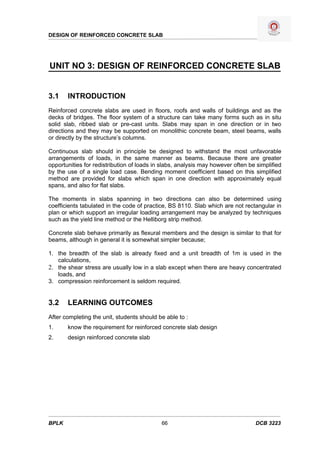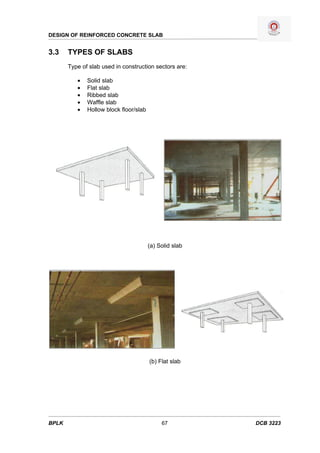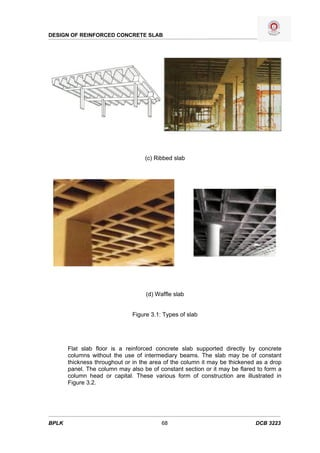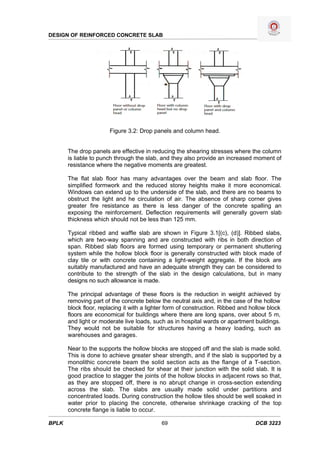Anúncio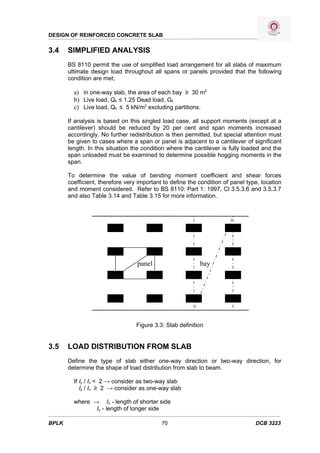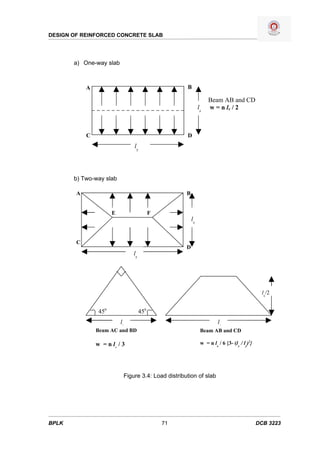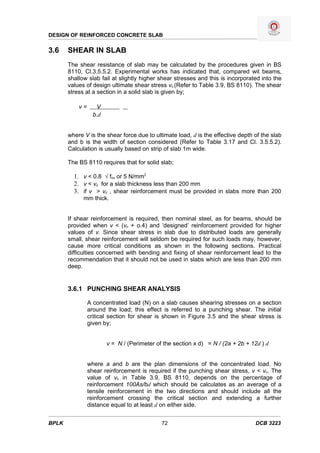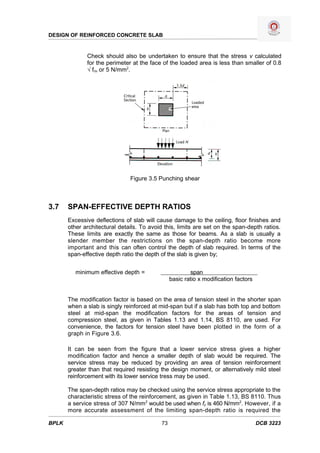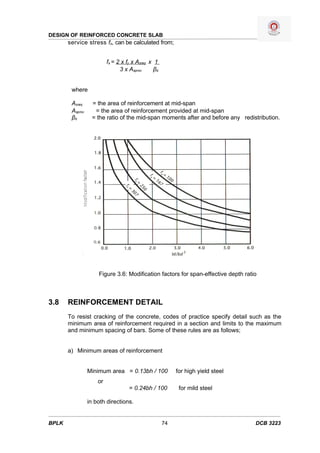Anúncio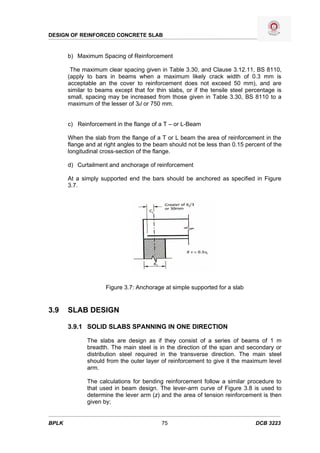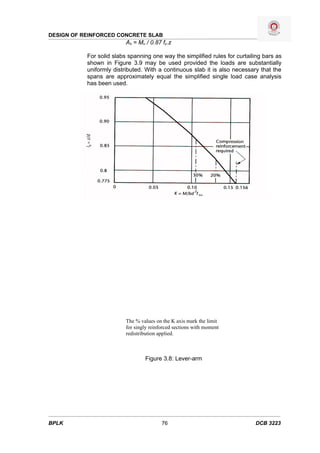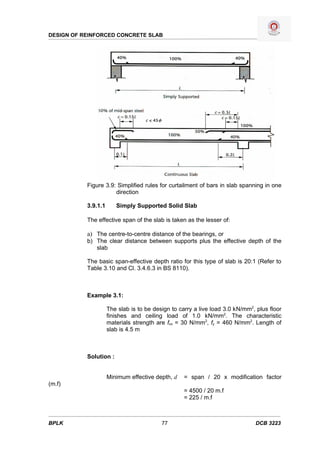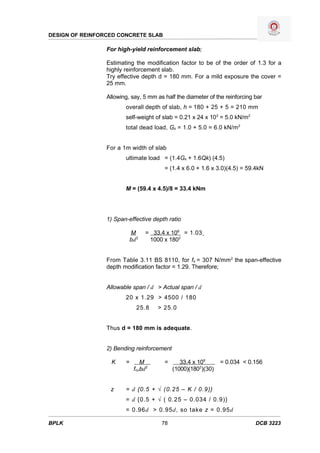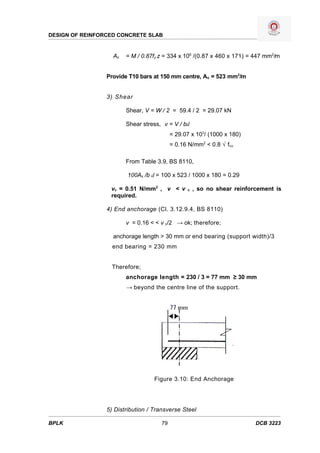Anúncio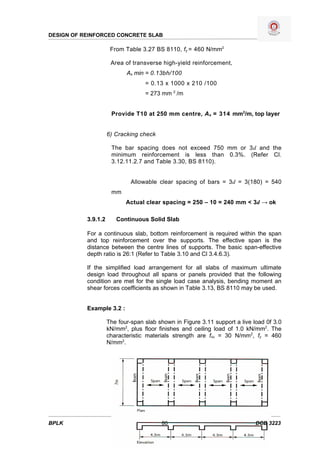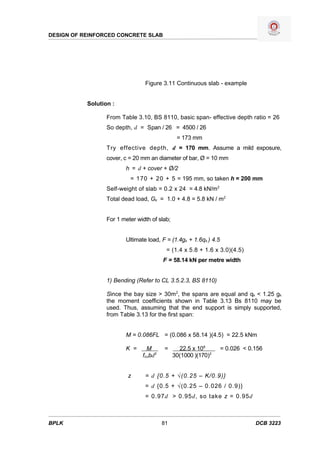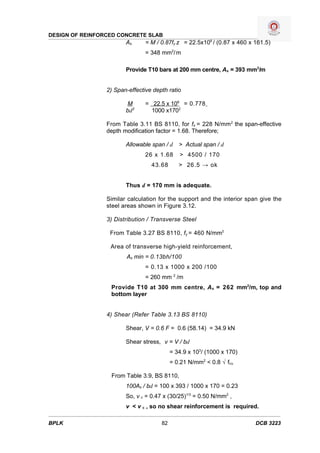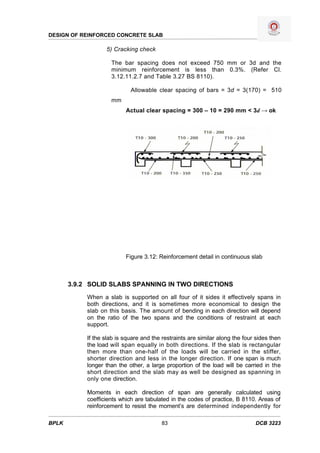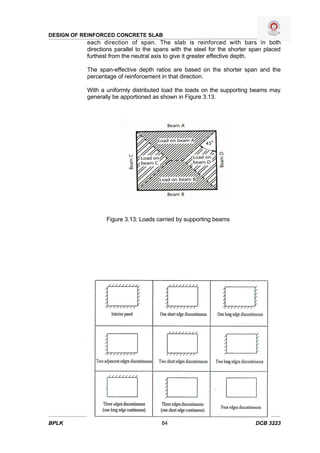Anúncio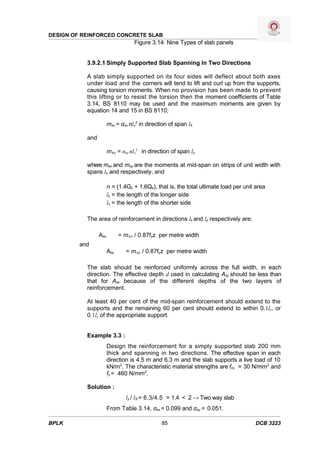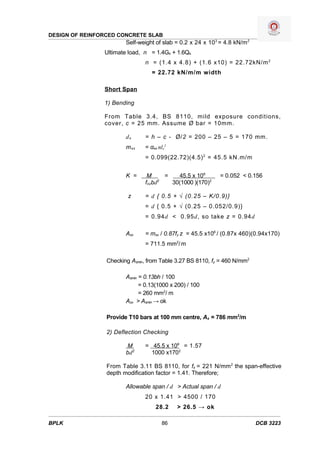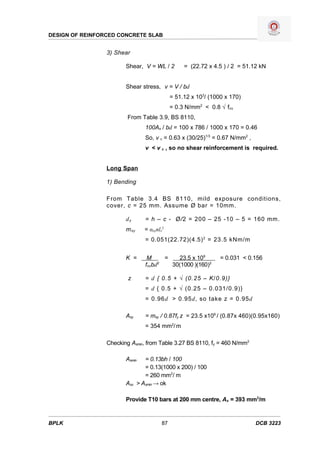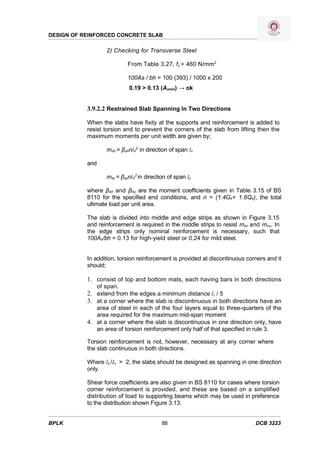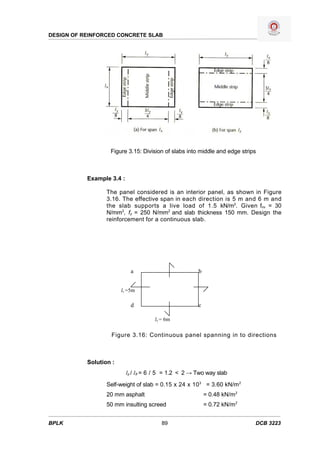Anúncio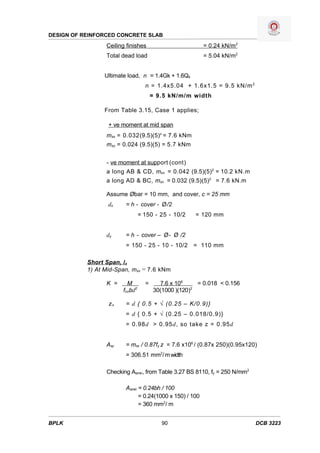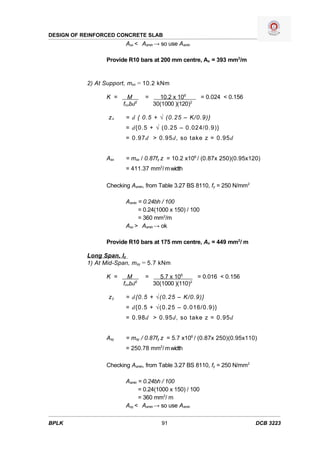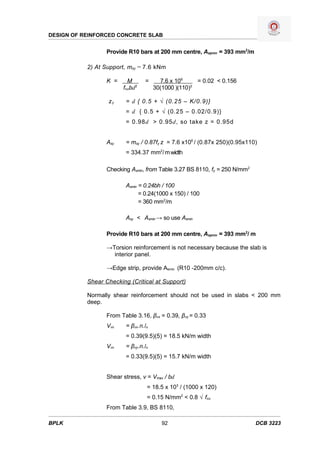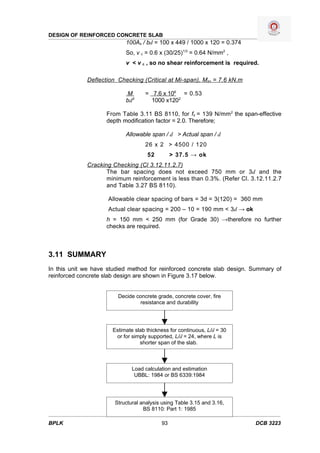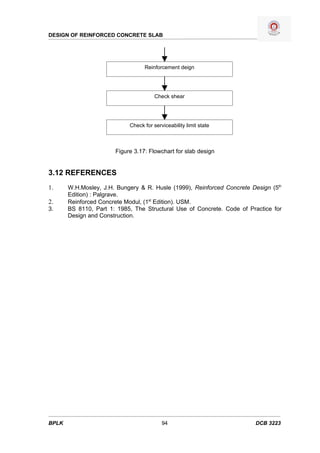Próximos SlideShares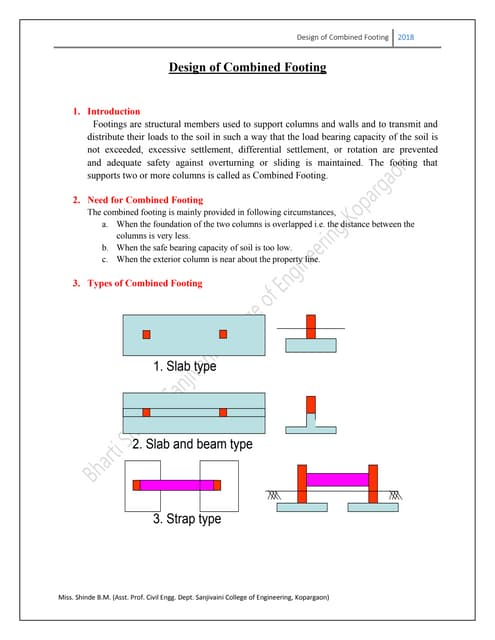Design of combined footing ppt
Carregando em ... 3
1 de 29
Anúncio

### Reinforced slab

1. DESIGN OF REINFORCED CONCRETE SLAB UNIT NO 3: DESIGN OF REINFORCED CONCRETE SLAB 3.1 INTRODUCTION Reinforced concrete slabs are used in floors, roofs and walls of buildings and as the decks of bridges. The floor system of a structure can take many forms such as in situ solid slab, ribbed slab or pre-cast units. Slabs may span in one direction or in two directions and they may be supported on monolithic concrete beam, steel beams, walls or directly by the structure’s columns. Continuous slab should in principle be designed to withstand the most unfavorable arrangements of loads, in the same manner as beams. Because there are greater opportunities for redistribution of loads in slabs, analysis may however often be simplified by the use of a single load case. Bending moment coefficient based on this simplified method are provided for slabs which span in one direction with approximately equal spans, and also for flat slabs. The moments in slabs spanning in two directions can also be determined using coefficients tabulated in the code of practice, BS 8110. Slab which are not rectangular in plan or which support an irregular loading arrangement may be analyzed by techniques such as the yield line method or the Helliborg strip method. Concrete slab behave primarily as flexural members and the design is similar to that for beams, although in general it is somewhat simpler because; 1. the breadth of the slab is already fixed and a unit breadth of 1m is used in the calculations, 2. the shear stress are usually low in a slab except when there are heavy concentrated loads, and 3. compression reinforcement is seldom required. 3.2 LEARNING OUTCOMES After completing the unit, students should be able to : 1. know the requirement for reinforced concrete slab design 2. design reinforced concrete slab BPLK 66 DCB 3223
2. DESIGN OF REINFORCED CONCRETE SLAB 3.3 TYPES OF SLABS Type of slab used in construction sectors are: • Solid slab • Flat slab • Ribbed slab • Waffle slab • Hollow block floor/slab (a) Solid slab (b) Flat slab BPLK 67 DCB 3223
3. DESIGN OF REINFORCED CONCRETE SLAB (c) Ribbed slab (d) Waffle slab Figure 3.1: Types of slab Flat slab floor is a reinforced concrete slab supported directly by concrete columns without the use of intermediary beams. The slab may be of constant thickness throughout or in the area of the column it may be thickened as a drop panel. The column may also be of constant section or it may be flared to form a column head or capital. These various form of construction are illustrated in Figure 3.2. BPLK 68 DCB 3223
6. DESIGN OF REINFORCED CONCRETE SLAB a) One-way slab A B Beam AB and CD lx w = n lx / 2 C D ly b) Two-way slab A B E F lx C D ly lx/2 450 450 lx lx Beam AC and BD Beam AB and CD w = n lx / 3 w = n lx / 6 {3- (lx / ly)2} Figure 3.4: Load distribution of slab BPLK 71 DCB 3223
7. DESIGN OF REINFORCED CONCRETE SLAB 3.6 SHEAR IN SLAB The shear resistance of slab may be calculated by the procedures given in BS 8110, Cl.3.5.5.2. Experimental works has indicated that, compared wit beams, shallow slab fail at slightly higher shear stresses and this is incorporated into the values of design ultimate shear stress vc.(Refer to Table 3.9, BS 8110). The shear stress at a section in a solid slab is given by; v= V b.d where V is the shear force due to ultimate load, d is the effective depth of the slab and b is the width of section considered (Refer to Table 3.17 and Cl. 3.5.5.2). Calculation is usually based on strip of slab 1m wide. The BS 8110 requires that for solid slab; 1. v < 0.8 √ fcu or 5 N/mm2 2. v < vc for a slab thickness less than 200 mm 3. if v > vc , shear reinforcement must be provided in slabs more than 200 mm thick. If shear reinforcement is required, then nominal steel, as for beams, should be provided when v < (vc + o.4) and ‘designed’ reinforcement provided for higher values of v. Since shear stress in slab due to distributed loads are generally small, shear reinforcement will seldom be required for such loads may, however, cause more critical conditions as shown in the following sections. Practical difficulties concerned with bending and fixing of shear reinforcement lead to the recommendation that it should not be used in slabs which are less than 200 mm deep. 3.6.1 PUNCHING SHEAR ANALYSIS A concentrated load (N) on a slab causes shearing stresses on a section around the load; this effect is referred to a punching shear. The initial critical section for shear is shown in Figure 3.5 and the shear stress is given by; v = N / (Perimeter of the section x d) = N / (2a + 2b + 12d ) d where a and b are the plan dimensions of the concentrated load. No shear reinforcement is required if the punching shear stress, v < vc. The value of vc in Table 3.9, BS 8110, depends on the percentage of reinforcement 100As/bd which should be calculates as an average of a tensile reinforcement in the two directions and should include all the reinforcement crossing the critical section and extending a further distance equal to at least d on either side. BPLK 72 DCB 3223
8. DESIGN OF REINFORCED CONCRETE SLAB Check should also be undertaken to ensure that the stress v calculated for the perimeter at the face of the loaded area is less than smaller of 0.8 √ fcu or 5 N/mm2. Figure 3.5 Punching shear 3.7 SPAN-EFFECTIVE DEPTH RATIOS Excessive deflections of slab will cause damage to the ceiling, floor finishes and other architectural details. To avoid this, limits are set on the span-depth ratios. These limits are exactly the same as those for beams. As a slab is usually a slender member the restrictions on the span-depth ratio become more important and this can often control the depth of slab required. In terms of the span-effective depth ratio the depth of the slab is given by; minimum effective depth = span basic ratio x modification factors The modification factor is based on the area of tension steel in the shorter span when a slab is singly reinforced at mid-span but if a slab has both top and bottom steel at mid-span the modification factors for the areas of tension and compression steel, as given in Tables 1.13 and 1.14, BS 8110, are used. For convenience, the factors for tension steel have been plotted in the form of a graph in Figure 3.6. It can be seen from the figure that a lower service stress gives a higher modification factor and hence a smaller depth of slab would be required. The service stress may be reduced by providing an area of tension reinforcement greater than that required resisting the design moment, or alternatively mild steel reinforcement with its lower service tress may be used. The span-depth ratios may be checked using the service stress appropriate to the characteristic stress of the reinforcement, as given in Table 1.13, BS 8110. Thus a service stress of 307 N/mm2 would be used when fy is 460 N/mm2. However, if a more accurate assessment of the limiting span-depth ratio is required the BPLK 73 DCB 3223
9. DESIGN OF REINFORCED CONCRETE SLAB service stress fs, can be calculated from; fs = 2 x fy x Asreq x 1 3 x Asprov βb where Asreq = the area of reinforcement at mid-span Asprov = the area of reinforcement provided at mid-span βb = the ratio of the mid-span moments after and before any redistribution. Figure 3.6: Modification factors for span-effective depth ratio 3.8 REINFORCEMENT DETAIL To resist cracking of the concrete, codes of practice specify detail such as the minimum area of reinforcement required in a section and limits to the maximum and minimum spacing of bars. Some of these rules are as follows; a) Minimum areas of reinforcement Minimum area = 0.13bh / 100 for high yield steel or = 0.24bh / 100 for mild steel in both directions. BPLK 74 DCB 3223
10. DESIGN OF REINFORCED CONCRETE SLAB b) Maximum Spacing of Reinforcement The maximum clear spacing given in Table 3.30, and Clause 3.12.11, BS 8110, (apply to bars in beams when a maximum likely crack width of 0.3 mm is acceptable an the cover to reinforcement does not exceed 50 mm), and are similar to beams except that for thin slabs, or if the tensile steel percentage is small, spacing may be increased from those given in Table 3.30, BS 8110 to a maximum of the lesser of 3d or 750 mm. c) Reinforcement in the flange of a T – or L-Beam When the slab from the flange of a T or L beam the area of reinforcement in the flange and at right angles to the beam should not be less than 0.15 percent of the longitudinal cross-section of the flange. d) Curtailment and anchorage of reinforcement At a simply supported end the bars should be anchored as specified in Figure 3.7. Figure 3.7: Anchorage at simple supported for a slab 3.9 SLAB DESIGN 3.9.1 SOLID SLABS SPANNING IN ONE DIRECTION The slabs are design as if they consist of a series of beams of 1 m breadth. The main steel is in the direction of the span and secondary or distribution steel required in the transverse direction. The main steel should from the outer layer of reinforcement to give it the maximum level arm. The calculations for bending reinforcement follow a similar procedure to that used in beam design. The lever-arm curve of Figure 3.8 is used to determine the lever arm (z) and the area of tension reinforcement is then given by; BPLK 75 DCB 3223
11. DESIGN OF REINFORCED CONCRETE SLAB As = Mu / 0.87 fy.z For solid slabs spanning one way the simplified rules for curtailing bars as shown in Figure 3.9 may be used provided the loads are substantially uniformly distributed. With a continuous slab it is also necessary that the spans are approximately equal the simplified single load case analysis has been used. The % values on the K axis mark the limit for singly reinforced sections with moment redistribution applied. Figure 3.8: Lever-arm BPLK 76 DCB 3223
12. DESIGN OF REINFORCED CONCRETE SLAB Figure 3.9: Simplified rules for curtailment of bars in slab spanning in one direction 3.9.1.1 Simply Supported Solid Slab The effective span of the slab is taken as the lesser of: a) The centre-to-centre distance of the bearings, or b) The clear distance between supports plus the effective depth of the slab The basic span-effective depth ratio for this type of slab is 20:1 (Refer to Table 3.10 and Cl. 3.4.6.3 in BS 8110). Example 3.1: The slab is to be design to carry a live load 3.0 kN/mm2, plus floor finishes and ceiling load of 1.0 kN/mm2. The characteristic materials strength are fcu = 30 N/mm2, fy = 460 N/mm2. Length of slab is 4.5 m Solution : Minimum effective depth, d = span / 20 x modification factor (m.f) = 4500 / 20 m.f = 225 / m.f BPLK 77 DCB 3223
13. DESIGN OF REINFORCED CONCRETE SLAB For high-yield reinforcement slab; Estimating the modification factor to be of the order of 1.3 for a highly reinforcement slab. Try effective depth d = 180 mm. For a mild exposure the cover = 25 mm. Allowing, say, 5 mm as half the diameter of the reinforcing bar overall depth of slab, h = 180 + 25 + 5 = 210 mm self-weight of slab = 0.21 x 24 x 103 = 5.0 kN/m2 total dead load, Gk = 1.0 + 5.0 = 6.0 kN/m2 For a 1m width of slab ultimate load = (1.4Gk + 1.6Qk) (4.5) = (1.4 x 6.0 + 1.6 x 3.0)(4.5) = 59.4kN M = (59.4 x 4.5)/8 = 33.4 kNm 1) Span-effective depth ratio M = 33.4 x 106 = 1.03 bd2 1000 x 1802 From Table 3.11 BS 8110, for fs = 307 N/mm 2 the span-effective depth modification factor = 1.29. Therefore; Allowable span / d > Actual span / d 20 x 1.29 > 4500 / 180 25.8 > 25.0 Thus d = 180 mm is adequate. 2) Bending reinforcement K = M = 33.4 x 106 = 0.034 < 0.156 fcubd2 (1000)(1802)(30) z = d {0.5 + √ (0.25 – K / 0.9)} = d {0.5 + √ ( 0.25 – 0.034 / 0.9)} = 0.96d > 0.95d, so take z = 0.95d BPLK 78 DCB 3223
14. DESIGN OF REINFORCED CONCRETE SLAB As = M / 0.87fy z = 334 x 106 /(0.87 x 460 x 171) = 447 mm2/m Provide T10 bars at 150 mm centre, As = 523 mm2/m 3) Shear Shear, V = W / 2 = 59.4 / 2 = 29.07 kN Shear stress, v = V / bd = 29.07 x 103/ (1000 x 180) = 0.16 N/mm2 < 0.8 √ fcu From Table 3.9, BS 8110, 100As /b d = 100 x 523 / 1000 x 180 = 0.29 vc = 0.51 N/mm2 , v < v c , so no shear reinforcement is required. 4) End anchorage (Cl. 3.12.9.4, BS 8110) v = 0.16 < < v c/2 → ok; therefore; anchorage length > 30 mm or end bearing (support width)/3 end bearing = 230 mm Therefore; anchorage length = 230 / 3 = 77 mm ≥ 30 mm → beyond the centre line of the support. Figure 3.10: End Anchorage 5) Distribution / Transverse Steel BPLK 79 DCB 3223
15. DESIGN OF REINFORCED CONCRETE SLAB From Table 3.27 BS 8110, fy = 460 N/mm2 Area of transverse high-yield reinforcement, As min = 0.13bh/100 = 0.13 x 1000 x 210 /100 = 273 mm 2 /m Provide T10 at 250 mm centre, A s = 314 mm2/m, top layer 6) Cracking check The bar spacing does not exceed 750 mm or 3d and the minimum reinforcement is less than 0.3%. (Refer Cl. 3.12.11.2.7 and Table 3.30, BS 8110). Allowable clear spacing of bars = 3d = 3(180) = 540 mm Actual clear spacing = 250 – 10 = 240 mm < 3d → ok 3.9.1.2 Continuous Solid Slab For a continuous slab, bottom reinforcement is required within the span and top reinforcement over the supports. The effective span is the distance between the centre lines of supports. The basic span-effective depth ratio is 26:1 (Refer to Table 3.10 and Cl 3.4.6.3). If the simplified load arrangement for all slabs of maximum ultimate design load throughout all spans or panels provided that the following condition are met for the single load case analysis, bending moment an shear forces coefficients as shown in Table 3.13, BS 8110 may be used. Example 3.2 : The four-span slab shown in Figure 3.11 support a live load 0f 3.0 kN/mm2, plus floor finishes and ceiling load of 1.0 kN/mm2. The characteristic materials strength are fcu = 30 N/mm2, fy = 460 N/mm2. BPLK 80 DCB 3223
16. DESIGN OF REINFORCED CONCRETE SLAB Figure 3.11 Continuous slab - example Solution : From Table 3.10, BS 8110, basic span- effective depth ratio = 26 So depth, d = Span / 26 = 4500 / 26 = 173 mm Try effective depth, d = 170 mm. Assume a mild exposure, cover, c = 20 mm an diameter of bar, Ø = 10 mm h = d + cover + Ø/2 = 170 + 20 + 5 = 195 mm, so taken h = 200 mm Self-weight of slab = 0.2 x 24 = 4.8 kN/m2 Total dead load, Gk = 1.0 + 4.8 = 5.8 kN / m2 For 1 meter width of slab; Ultimate load, F = (1.4gk + 1.6qk ) 4.5 = (1.4 x 5.8 + 1.6 x 3.0)(4.5) F = 58.14 kN per metre width 1) Bending (Refer to CL 3.5.2.3, BS 8110) Since the bay size > 30m2, the spans are equal and qk < 1.25 gk the moment coefficients shown in Table 3.13 Bs 8110 may be used. Thus, assuming that the end support is simply supported, from Table 3.13 for the first span: M = 0.086FL = (0.086 x 58.14 )(4.5) = 22.5 kNm K = M = 22.5 x 106 = 0.026 < 0.156 fcubd2 30(1000 )(170)2 z = d {0.5 + √(0.25 – K/0.9)} = d {0.5 + √(0.25 – 0.026 / 0.9)} = 0.97d > 0.95d, so take z = 0.95d BPLK 81 DCB 3223
17. DESIGN OF REINFORCED CONCRETE SLAB As = M / 0.87fy z = 22.5x106 / (0.87 x 460 x 161.5) = 348 mm2/ m Provide T10 bars at 200 mm centre, As = 393 mm2/m 2) Span-effective depth ratio M = 22.5 x 106 = 0.778 bd2 1000 x1702 From Table 3.11 BS 8110, for fs = 228 N/mm 2 the span-effective depth modification factor = 1.68. Therefore; Allowable span / d > Actual span / d 26 x 1.68 > 4500 / 170 43.68 > 26.5 → ok Thus d = 170 mm is adequate. Similar calculation for the support and the interior span give the steel areas shown in Figure 3.12. 3) Distribution / Transverse Steel From Table 3.27 BS 8110, fy = 460 N/mm2 Area of transverse high-yield reinforcement, As min = 0.13bh/100 = 0.13 x 1000 x 200 /100 = 260 mm 2 /m Provide T10 at 300 mm centre, A s = 262 mm2/m, top and bottom layer 4) Shear (Refer Table 3.13 BS 8110) Shear, V = 0.6 F = 0.6 (58.14) = 34.9 kN Shear stress, v = V / bd = 34.9 x 103/ (1000 x 170) = 0.21 N/mm2 < 0.8 √ fcu From Table 3.9, BS 8110, 100As / bd = 100 x 393 / 1000 x 170 = 0.23 So, v c = 0.47 x (30/25)1/3 = 0.50 N/mm2 , v < v c , so no shear reinforcement is required. BPLK 82 DCB 3223
18. DESIGN OF REINFORCED CONCRETE SLAB 5) Cracking check The bar spacing does not exceed 750 mm or 3d and the minimum reinforcement is less than 0.3%. (Refer Cl. 3.12.11.2.7 and Table 3.27 BS 8110). Allowable clear spacing of bars = 3d = 3(170) = 510 mm Actual clear spacing = 300 – 10 = 290 mm < 3d → ok Figure 3.12: Reinforcement detail in continuous slab 3.9.2 SOLID SLABS SPANNING IN TWO DIRECTIONS When a slab is supported on all four of it sides it effectively spans in both directions, and it is sometimes more economical to design the slab on this basis. The amount of bending in each direction will depend on the ratio of the two spans and the conditions of restraint at each support. If the slab is square and the restraints are similar along the four sides then the load will span equally in both directions. If the slab is rectangular then more than one-half of the loads will be carried in the stiffer, shorter direction and less in the longer direction. If one span is much longer than the other, a large proportion of the load will be carried in the short direction and the slab may as well be designed as spanning in only one direction. Moments in each direction of span are generally calculated using coefficients which are tabulated in the codes of practice, B 8110. Areas of reinforcement to resist the moment’s are determined independently for BPLK 83 DCB 3223
19. DESIGN OF REINFORCED CONCRETE SLAB each direction of span. The slab is reinforced with bars in both directions parallel to the spans with the steel for the shorter span placed furthest from the neutral axis to give it greater effective depth. The span-effective depth ratios are based on the shorter span and the percentage of reinforcement in that direction. With a uniformly distributed load the loads on the supporting beams may generally be apportioned as shown in Figure 3.13. Figure 3.13: Loads carried by supporting beams BPLK 84 DCB 3223
20. DESIGN OF REINFORCED CONCRETE SLAB Figure 3.14: Nine Types of slab panels 3.9.2.1 Simply Supported Slab Spanning In Two Directions A slab simply supported on its four sides will deflect about both axes under load and the corners will tend to lift and curl up from the supports, causing torsion moments. When no provision has been made to prevent this lifting or to resist the torsion then the moment coefficients of Table 3.14, BS 8110 may be used and the maximum moments are given by equation 14 and 15 in BS 8110; msx = αsx nlx2 in direction of span lX and m sy = αsy nlx2 in direction of span ly where msx and msy are the moments at mid-span on strips of unit width with spans lx and respectively, and n = (1.4Gk + 1.6Qk), that is, the total ultimate load per unit area ly = the length of the longer side lx = the length of the shorter side The area of reinforcement in directions lx and ly respectively are; Asx = m sx / 0.87fyz per metre width and Asy = m sy / 0.87fyz per metre width The slab should be reinforced uniformly across the full width, in each direction. The effective depth d used in calculating Asy should be less than that for Asx because of the different depths of the two layers of reinforcement. At least 40 per cent of the mid-span reinforcement should extend to the supports and the remaining 60 per cent should extend to within 0.1lx, or 0.1ly of the appropriate support. Example 3.3 : Design the reinforcement for a simply supported slab 200 mm thick and spanning in two directions. The effective span in each direction is 4.5 m and 6.3 m and the slab supports a live load of 10 kN/m2. The characteristic material strengths are fcu = 30 N/mm2 and fy = 460 N/mm2. Solution : ly / lX = 6.3/4.5 = 1.4 < 2 → Two way slab From Table 3.14, αsx = 0.099 and αsy = 0.051. BPLK 85 DCB 3223
21. DESIGN OF REINFORCED CONCRETE SLAB Self-weight of slab = 0.2 x 24 x 103 = 4.8 kN/m2 Ultimate load, n = 1.4Gk + 1.6Qk n = (1.4 x 4.8) + (1.6 x10) = 22.72kN/m 2 = 22.72 kN/m/m width Short Span 1) Bending From Table 3.4, BS 8110, mild exposure conditions, cover, c = 25 mm. Assume Ø bar = 10mm. dx = h – c - Ø/2 = 200 – 25 – 5 = 170 mm. m sx = αsx nlx2 = 0.099(22.72)(4.5) 2 = 45.5 kN.m/m K = M = 45.5 x 106 = 0.052 < 0.156 2 fcubd 30(1000 )(170)2 z = d { 0.5 + √ (0.25 – K/0.9)} = d { 0.5 + √ (0.25 – 0.052/0.9)} = 0.94d < 0.95d, so take z = 0.94d Asx = msx / 0.87fy z = 45.5 x106 / (0.87x 460)(0.94x170) = 711.5 mm2/ m Checking Asmin, from Table 3.27 BS 8110, fy = 460 N/mm2 Asmin = 0.13bh / 100 = 0.13(1000 x 200) / 100 = 260 mm2/ m Asx > Asmin → ok Provide T10 bars at 100 mm centre, As = 786 mm2/m 2) Deflection Checking M = 45.5 x 106 = 1.57 bd2 1000 x1702 From Table 3.11 BS 8110, for fs = 221 N/mm 2 the span-effective depth modification factor = 1.41. Therefore; Allowable span / d > Actual span / d 20 x 1.41 > 4500 / 170 28.2 > 26.5 → ok BPLK 86 DCB 3223
22. DESIGN OF REINFORCED CONCRETE SLAB 3) Shear Shear, V = WL / 2 = (22.72 x 4.5 ) / 2 = 51.12 kN Shear stress, v = V / bd = 51.12 x 103/ (1000 x 170) = 0.3 N/mm2 < 0.8 √ fcu From Table 3.9, BS 8110, 100As / bd = 100 x 786 / 1000 x 170 = 0.46 So, v c = 0.63 x (30/25)1/3 = 0.67 N/mm2 , v < v c , so no shear reinforcement is required. Long Span 1) Bending From Table 3.4 BS 8110, mild exposure conditions, cover, c = 25 mm. Assume Ø bar = 10mm. dy = h – c - Ø/2 = 200 – 25 -10 – 5 = 160 mm. m sy = αsynlx2 = 0.051(22.72)(4.5) 2 = 23.5 kNm/m K = M = 23.5 x 106 = 0.031 < 0.156 2 fcubd 30(1000 )(160)2 z = d { 0.5 + √ (0.25 – K/0.9)} = d { 0.5 + √ (0.25 – 0.031/0.9)} = 0.96d > 0.95d, so take z = 0.95d Asy = msy / 0.87fy z = 23.5 x106 / (0.87x 460)(0.95x160) = 354 mm2/ m Checking Asmin, from Table 3.27 BS 8110, fy = 460 N/mm2 Asmin = 0.13bh / 100 = 0.13(1000 x 200) / 100 = 260 mm2/ m Asx > Asmin → ok Provide T10 bars at 200 mm centre, As = 393 mm2/m BPLK 87 DCB 3223
23. DESIGN OF REINFORCED CONCRETE SLAB 2) Checking for Transverse Steel From Table 3.27, fy = 460 N/mm2 100As / bh = 100 (393) / 1000 x 200 0.19 > 0.13 (Asmin) → ok 3.9.2.2 Restrained Slab Spanning In Two Directions When the slabs have fixity at the supports and reinforcement is added to resist torsion and to prevent the corners of the slab from lifting then the maximum moments per unit width are given by; msX = βsXnlX2 in direction of span lx and msy = βsynlX2 in direction of span ly where βsX and βSy are the moment coefficients given in Table 3.15 of BS 8110 for the specified end conditions, and n = (1.4Gk+ 1.6Qk), the total ultimate load per unit area. The slab is divided into middle and edge strips as shown in Figure 3.15 and reinforcement is required in the middle strips to resist msx and msy, In the edge strips only nominal reinforcement is necessary, such that 100As/bh = 0.13 for high-yield steel or 0.24 for mild steel. In addition, torsion reinforcement is provided at discontinuous corners and it should; 1. consist of top and bottom mats, each having bars in both directions of span. 2. extend from the edges a minimum distance lx / 5 3. at a corner where the slab is discontinuous in both directions have an area of steel in each of the four layers equal to three-quarters of the area required for the maximum mid-span moment 4. at a corner where the slab is discontinuous in one direction only, have an area of torsion reinforcement only half of that specified in rule 3. Torsion reinforcement is not, however, necessary at any corner where the slab continuous in both directions. Where ly /Ix > 2, the slabs should be designed as spanning in one direction only. Shear force coefficients are also given in BS 8110 for cases where torsion corner reinforcement is provided, and these are based on a simplified distribution of load to supporting beams which may be used in preference to the distribution shown Figure 3.13. BPLK 88 DCB 3223
24. DESIGN OF REINFORCED CONCRETE SLAB Figure 3.15: Division of slabs into middle and edge strips Example 3.4 : The panel considered is an interior panel, as shown in Figure 3.16. The effective span in each direction is 5 m and 6 m and the slab supports a live load of 1.5 kN/m2. Given fcu = 30 N/mm2, fy = 250 N/mm2 and slab thickness 150 mm. Design the reinforcement for a continuous slab. a b lx =5m d c ly = 6m Figure 3.16: Continuous panel spanning in to directions Solution : ly / lX = 6 / 5 = 1.2 < 2 → Two way slab Self-weight of slab = 0.15 x 24 x 103 = 3.60 kN/m2 20 mm asphalt = 0.48 kN/m2 50 mm insulting screed = 0.72 kN/m2 BPLK 89 DCB 3223
25. DESIGN OF REINFORCED CONCRETE SLAB Ceiling finishes = 0.24 kN/m2 Total dead load = 5.04 kN/m2 Ultimate load, n = 1.4Gk + 1.6Qk n = 1.4x5.04 + 1.6x1.5 = 9.5 kN/m 2 = 9.5 kN/m/m width From Table 3.15, Case 1 applies; + ve moment at mid span msx = 0.032(9.5)(5)z = 7.6 kNm msy = 0.024 (9.5)(5) = 5.7 kNm - ve moment at support (cont) a long AB & CD, msx = 0.042 (9.5)(5)2 = 10.2 kN.m a long AD & BC, msx = 0.032 (9.5)(5)2 = 7.6 kN.m Assume Øbar = 10 mm, and cover, c = 25 mm dx = h - cover - Ø /2 = 150 - 25 - 10/2 = 120 mm dy = h - cover – Ø- Ø /2 = 150 - 25 - 10 - 10/2 = 110 mm Short Span, lx 1) At Mid-Span, msx = 7.6 kNm K = M = 7.6 x 106 = 0.018 < 0.156 fcubd2 30(1000 )(120)2 zx = d { 0.5 + √ (0.25 – K/0.9)} = d { 0.5 + √ (0.25 – 0.018/0.9)} = 0.98d > 0.95d, so take z = 0.95d Asy = msx / 0.87fy z = 7.6 x106 / (0.87x 250)(0.95x120) = 306.51 mm2/ m width Checking Asmin, from Table 3.27 BS 8110, fy = 250 N/mm2 Asmin = 0.24bh / 100 = 0.24(1000 x 150) / 100 = 360 mm2/ m BPLK 90 DCB 3223
26. DESIGN OF REINFORCED CONCRETE SLAB Asx < Asmin → so use Asmin Provide R10 bars at 200 mm centre, As = 393 mm2/m 2) At Support, msx = 10.2 kNm K = M = 10.2 x 106 = 0.024 < 0.156 fcubd2 30(1000 )(120)2 zx = d { 0.5 + √ (0.25 – K/0.9)} = d{0.5 + √ (0.25 – 0.024/0.9)} = 0.97d > 0.95d, so take z = 0.95d Asx = msx / 0.87fy z = 10.2 x106 / (0.87x 250)(0.95x120) = 411.37 mm2/ m width Checking Asmin, from Table 3.27 BS 8110, fy = 250 N/mm2 Asmin = 0.24bh / 100 = 0.24(1000 x 150) / 100 = 360 mm2/m Asx > Asmin → ok Provide R10 bars at 175 mm centre, As = 449 mm2/ m Long Span, ly 1) At Mid-Span, msy = 5.7 kNm K = M = 5.7 x 106 = 0.016 < 0.156 fcubd2 30(1000 )(110)2 zy = d{0.5 + √(0.25 – K/0.9)} = d{0.5 + √(0.25 – 0.016/0.9)} = 0.98d > 0.95d, so take z = 0.95d Asy = msy / 0.87fy z = 5.7 x106 / (0.87x 250)(0.95x110) = 250.78 mm2/ m width Checking Asmin, from Table 3.27 BS 8110, fy = 250 N/mm2 Asmin = 0.24bh / 100 = 0.24(1000 x 150) / 100 = 360 mm2/ m Asy < Asmin → so use Asmin BPLK 91 DCB 3223
27. DESIGN OF REINFORCED CONCRETE SLAB Provide R10 bars at 200 mm centre, Asprov = 393 mm2/m 2) At Support, msy = 7.6 kNm K = M = 7.6 x 106 = 0.02 < 0.156 fcubd2 30(1000 )(110)2 zy = d { 0.5 + √ (0.25 – K/0.9)} = d { 0.5 + √ (0.25 – 0.02/0.9)} = 0.98d > 0.95d, so take z = 0.95d Asy = msy / 0.87fy z = 7.6 x106 / (0.87x 250)(0.95x110) = 334.37 mm2/ m width Checking Asmin, from Table 3.27 BS 8110, fy = 250 N/mm2 Asmin = 0.24bh / 100 = 0.24(1000 x 150) / 100 = 360 mm2/m Asy < Asmin → so use Asmin Provide R10 bars at 200 mm centre, Asprov = 393 mm2/ m →Torsion reinforcement is not necessary because the slab is interior panel. →Edge strip, provide Asmin (R10 -200mm c/c). Shear Checking (Critical at Support) Normally shear reinforcement should not be used in slabs < 200 mm deep. From Table 3.16, βvx = 0.39, βvy = 0.33 Vvx = βvx.n.lx = 0.39(9.5)(5) = 18.5 kN/m width Vvx = βvy.n.lx = 0.33(9.5)(5) = 15.7 kN/m width Shear stress, v = Vmax / bd = 18.5 x 103 / (1000 x 120) = 0.15 N/mm2 < 0.8 √ fcu From Table 3.9, BS 8110, BPLK 92 DCB 3223
28. DESIGN OF REINFORCED CONCRETE SLAB 100As / bd = 100 x 449 / 1000 x 120 = 0.374 So, v c = 0.6 x (30/25)1/3 = 0.64 N/mm2 , v < v c , so no shear reinforcement is required. Deflection Checking (Critical at Mi-span), Msx = 7.6 kN.m M = 7.6 x 106 = 0.53 bd2 1000 x1202 From Table 3.11 BS 8110, for fs = 139 N/mm 2 the span-effective depth modification factor = 2.0. Therefore; Allowable span / d > Actual span / d 26 x 2 > 4500 / 120 52 > 37.5 → ok Cracking Checking (Cl 3.12.11.2.7) The bar spacing does not exceed 750 mm or 3d and the minimum reinforcement is less than 0.3%. (Refer Cl. 3.12.11.2.7 and Table 3.27 BS 8110). Allowable clear spacing of bars = 3d = 3(120) = 360 mm Actual clear spacing = 200 – 10 = 190 mm < 3d → ok h = 150 mm < 250 mm (for Grade 30) →therefore no further checks are required. 3.11 SUMMARY In this unit we have studied method for reinforced concrete slab design. Summary of reinforced concrete slab design are shown in Figure 3.17 below. Decide concrete grade, concrete cover, fire resistance and durability Estimate slab thickness for continuous, L/d = 30 or for simply supported, L/d = 24, where L is shorter span of the slab. Load calculation and estimation UBBL: 1984 or BS 6339:1984 Structural analysis using Table 3.15 and 3.16, BS 8110: Part 1: 1985 BPLK 93 DCB 3223
29. DESIGN OF REINFORCED CONCRETE SLAB Reinforcement deign Check shear Check for serviceability limit state Figure 3.17: Flowchart for slab design 3.12 REFERENCES 1. W.H.Mosley, J.H. Bungery & R. Husle (1999), Reinforced Concrete Design (5th Edition) : Palgrave. 2. Reinforced Concrete Modul, (1st Edition). USM. 3. BS 8110, Part 1: 1985, The Structural Use of Concrete. Code of Practice for Design and Construction. BPLK 94 DCB 3223
Anúncio